Publicité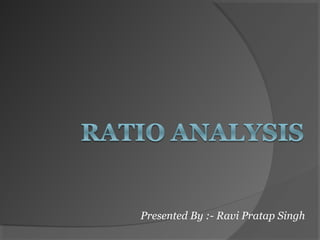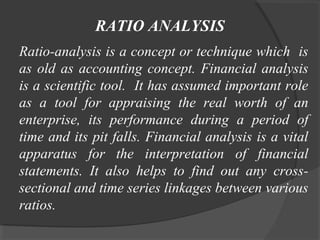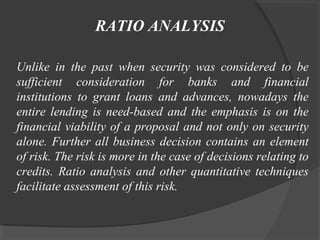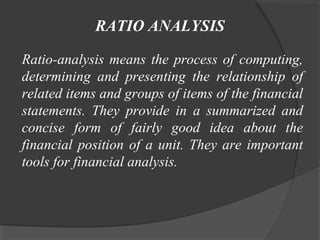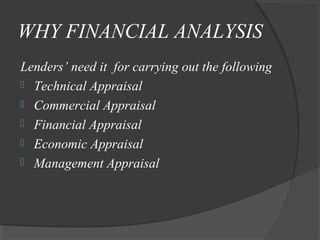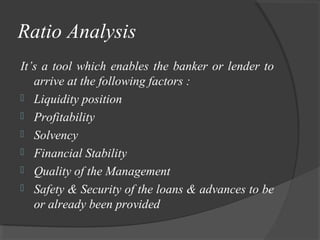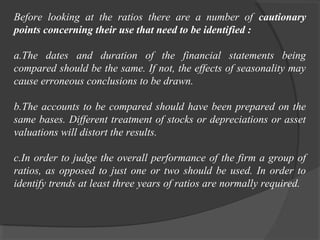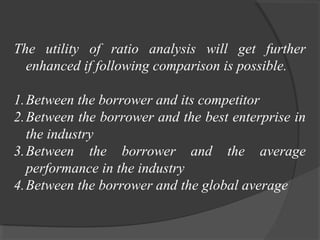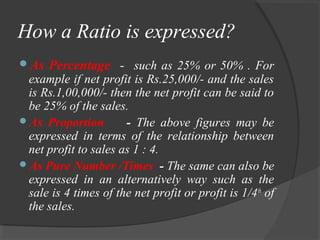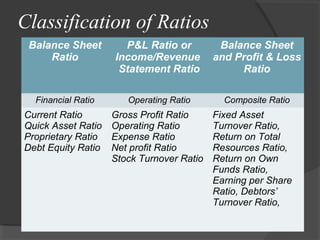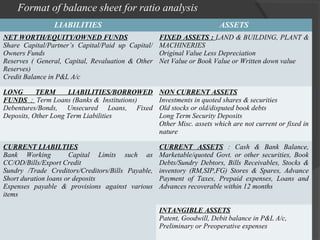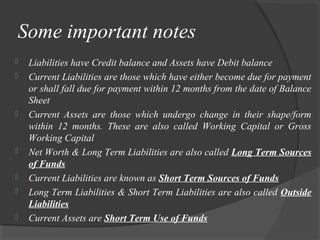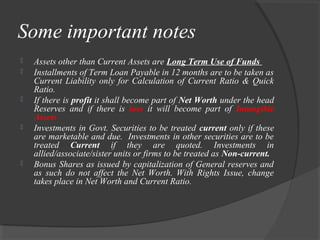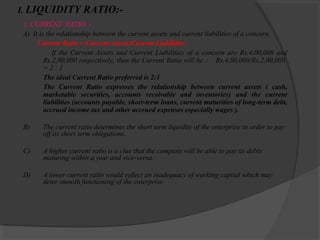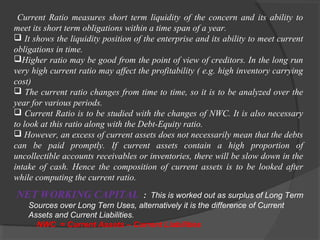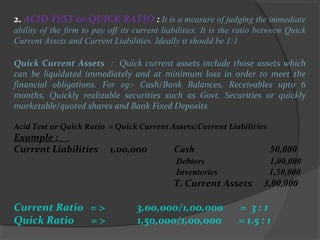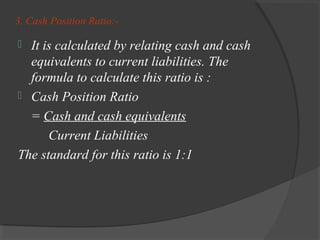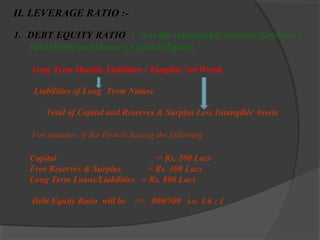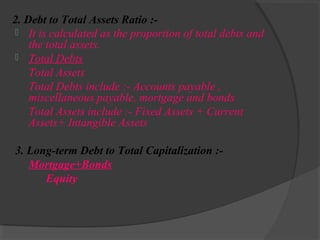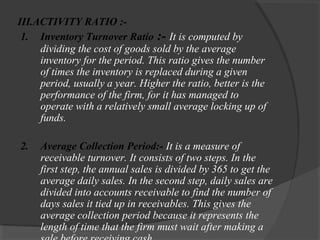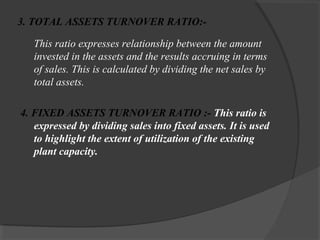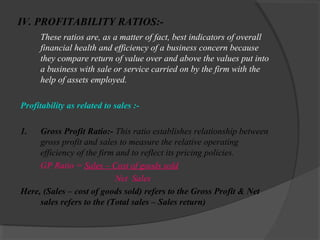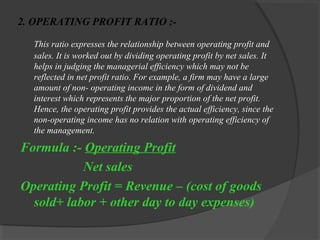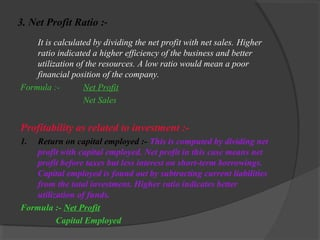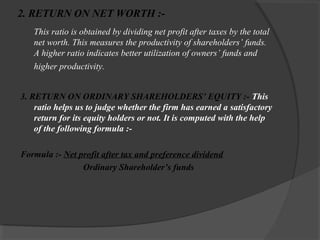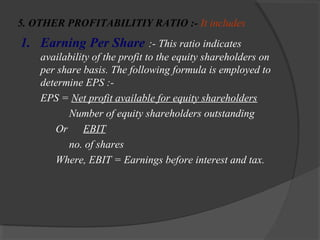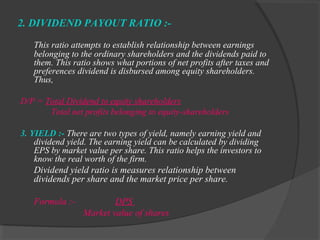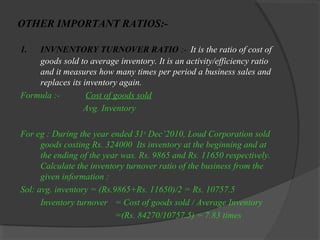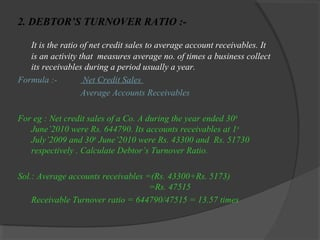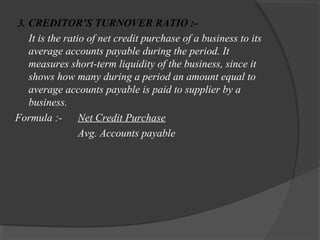1 sur 30
Publicité

### Ratio Analysis By- Ravi Thakur From CMD

1. Presented By :- Ravi Pratap Singh
2. RATIO ANALYSIS Ratio-analysis is a concept or technique which is as old as accounting concept. Financial analysis is a scientific tool. It has assumed important role as a tool for appraising the real worth of an enterprise, its performance during a period of time and its pit falls. Financial analysis is a vital apparatus for the interpretation of financial statements. It also helps to find out any crosssectional and time series linkages between various ratios.
3. RATIO ANALYSIS Unlike in the past when security was considered to be sufficient consideration for banks and financial institutions to grant loans and advances, nowadays the entire lending is need-based and the emphasis is on the financial viability of a proposal and not only on security alone. Further all business decision contains an element of risk. The risk is more in the case of decisions relating to credits. Ratio analysis and other quantitative techniques facilitate assessment of this risk.
4. RATIO ANALYSIS Ratio-analysis means the process of computing, determining and presenting the relationship of related items and groups of items of the financial statements. They provide in a summarized and concise form of fairly good idea about the financial position of a unit. They are important tools for financial analysis.
5. WHY FINANCIAL ANALYSIS Lenders’ need it for carrying out the following  Technical Appraisal  Commercial Appraisal  Financial Appraisal  Economic Appraisal  Management Appraisal
6. Ratio Analysis It’s a tool which enables the banker or lender to arrive at the following factors :  Liquidity position  Profitability  Solvency  Financial Stability  Quality of the Management  Safety & Security of the loans & advances to be or already been provided
7. Before looking at the ratios there are a number of cautionary points concerning their use that need to be identified : a.The dates and duration of the financial statements being compared should be the same. If not, the effects of seasonality may cause erroneous conclusions to be drawn. b.The accounts to be compared should have been prepared on the same bases. Different treatment of stocks or depreciations or asset valuations will distort the results. c.In order to judge the overall performance of the firm a group of ratios, as opposed to just one or two should be used. In order to identify trends at least three years of ratios are normally required.
8. The utility of ratio analysis will get further enhanced if following comparison is possible. 1.Between the borrower and its competitor 2.Between the borrower and the best enterprise in the industry 3.Between the borrower and the average performance in the industry 4.Between the borrower and the global average
9. How a Ratio is expressed? As Percentage - such as 25% or 50% . For example if net profit is Rs.25,000/- and the sales is Rs.1,00,000/- then the net profit can be said to be 25% of the sales. As Proportion - The above figures may be expressed in terms of the relationship between net profit to sales as 1 : 4. As Pure Number /Times - The same can also be expressed in an alternatively way such as the sale is 4 times of the net profit or profit is 1/4th of the sales.
10. Classification of Ratios Balance Sheet Ratio P&L Ratio or Income/Revenue Statement Ratio Balance Sheet and Profit & Loss Ratio Financial Ratio Operating Ratio Composite Ratio Current Ratio Quick Asset Ratio Proprietary Ratio Debt Equity Ratio Gross Profit Ratio Operating Ratio Expense Ratio Net profit Ratio Stock Turnover Ratio Fixed Asset Turnover Ratio, Return on Total Resources Ratio, Return on Own Funds Ratio, Earning per Share Ratio, Debtors’ Turnover Ratio,
11. Format of balance sheet for ratio analysis LIABILITIES ASSETS NET WORTH/EQUITY/OWNED FUNDS Share Capital/Partner’s Capital/Paid up Capital/ Owners Funds Reserves ( General, Capital, Revaluation & Other Reserves) Credit Balance in P&L A/c FIXED ASSETS : LAND & BUILDING, PLANT & MACHINERIES Original Value Less Depreciation Net Value or Book Value or Written down value LONG TERM LIABILITIES/BORROWED FUNDS : Term Loans (Banks & Institutions) Debentures/Bonds, Unsecured Loans, Fixed Deposits, Other Long Term Liabilities NON CURRENT ASSETS Investments in quoted shares & securities Old stocks or old/disputed book debts Long Term Security Deposits Other Misc. assets which are not current or fixed in nature CURRENT LIABILTIES Bank Working Capital Limits such as CC/OD/Bills/Export Credit Sundry /Trade Creditors/Creditors/Bills Payable, Short duration loans or deposits Expenses payable & provisions against various items CURRENT ASSETS : Cash & Bank Balance, Marketable/quoted Govt. or other securities, Book Debts/Sundry Debtors, Bills Receivables, Stocks & inventory (RM,SIP,FG) Stores & Spares, Advance Payment of Taxes, Prepaid expenses, Loans and Advances recoverable within 12 months INTANGIBLE ASSETS Patent, Goodwill, Debit balance in P&L A/c, Preliminary or Preoperative expenses
12. Some important notes        Liabilities have Credit balance and Assets have Debit balance Current Liabilities are those which have either become due for payment or shall fall due for payment within 12 months from the date of Balance Sheet Current Assets are those which undergo change in their shape/form within 12 months. These are also called Working Capital or Gross Working Capital Net Worth & Long Term Liabilities are also called Long Term Sources of Funds Current Liabilities are known as Short Term Sources of Funds Long Term Liabilities & Short Term Liabilities are also called Outside Liabilities Current Assets are Short Term Use of Funds
13. Some important notes      Assets other than Current Assets are Long Term Use of Funds Installments of Term Loan Payable in 12 months are to be taken as Current Liability only for Calculation of Current Ratio & Quick Ratio. If there is profit it shall become part of Net Worth under the head Reserves and if there is loss it will become part of Intangible Assets Investments in Govt. Securities to be treated current only if these are marketable and due. Investments in other securities are to be treated Current if they are quoted. Investments in allied/associate/sister units or firms to be treated as Non-current. Bonus Shares as issued by capitalization of General reserves and as such do not affect the Net Worth. With Rights Issue, change takes place in Net Worth and Current Ratio.
14. I. LIQUIDITY RATIO:- 1. CURRENT RATIO :A) It is the relationship between the current assets and current liabilities of a concern. Current Ratio = Current Assets/Current Liabilities If the Current Assets and Current Liabilities of a concern are Rs.4,00,000 and Rs.2,00,000 respectively, then the Current Ratio will be : Rs.4,00,000/Rs.2,00,000 =2:1 The ideal Current Ratio preferred is 2:1 The Current Ratio expresses the relationship between current assets ( cash, marketable securities, accounts receivable and inventories) and the current liabilities (accounts payable, short-term loans, current maturities of long-term debt, accrued income tax and other accrued expenses especially wages ). B) The current ratio determines the short term liquidity of the enterprise in order to pay off its short term obligations. C) A higher current ratio is a clue that the company will be able to pay its debts maturing within a year and vice-versa. D) A lower current ratio would reflect an inadequacy of working capital which may deter smooth functioning of the enterprise.
15. Current Ratio measures short term liquidity of the concern and its ability to meet its short term obligations within a time span of a year.  It shows the liquidity position of the enterprise and its ability to meet current obligations in time. Higher ratio may be good from the point of view of creditors. In the long run very high current ratio may affect the profitability ( e.g. high inventory carrying cost)  The current ratio changes from time to time, so it is to be analyzed over the year for various periods.  Current Ratio is to be studied with the changes of NWC. It is also necessary to look at this ratio along with the Debt-Equity ratio.  However, an excess of current assets does not necessarily mean that the debts can be paid promptly. If current assets contain a high proportion of uncollectible accounts receivables or inventories, there will be slow down in the intake of cash. Hence the composition of current assets is to be looked after while computing the current ratio. NET WORKING CAPITAL : This is worked out as surplus of Long Term Sources over Long Tern Uses, alternatively it is the difference of Current Assets and Current Liabilities. NWC = Current Assets – Current Liabilities
16. 2. ACID TEST or QUICK RATIO : It is a measure of judging the immediate ability of the firm to pay off its current liabilities. It is the ratio between Quick Current Assets and Current Liabilities. Ideally it should be 1:1 Quick Current Assets : Quick current assets include those assets which can be liquidated immediately and at minimum loss in order to meet the financial obligations. For eg:- Cash/Bank Balances, Receivables upto 6 months, Quickly realizable securities such as Govt. Securities or quickly marketable/quoted shares and Bank Fixed Deposits Acid Test or Quick Ratio = Quick Current Assets/Current Liabilities Example : Current Liabilities 1,00,000 Cash 50,000 Debtors Inventories 1,00,000 1,50,000 T. Current Assets Current Ratio = > Quick Ratio => 3,00,000/1,00,000 1,50,000/1,00,000 3,00,000 = 3:1 = 1.5 : 1
17. 3. Cash Position Ratio:- It is calculated by relating cash and cash equivalents to current liabilities. The formula to calculate this ratio is :  Cash Position Ratio = Cash and cash equivalents Current Liabilities The standard for this ratio is 1:1 
18. II. LEVERAGE RATIO :1. DEBT EQUITY RATIO : It is the relationship between borrower’s fund (Debt) and Owner’s Capital (Equity). Long Term Outside Liabilities / Tangible Net Worth Liabilities of Long Term Nature Total of Capital and Reserves & Surplus Less Intangible Assets For instance, if the Firm is having the following : Capital = Rs. 200 Lacs Free Reserves & Surplus = Rs. 300 Lacs Long Term Loans/Liabilities = Rs. 800 Lacs Debt Equity Ratio will be => 800/500 i.e. 1.6 : 1
19. 2. Debt to Total Assets Ratio : It is calculated as the proportion of total debts and the total assets.  Total Debts Total Assets Total Debts include :- Accounts payable , miscellaneous payable, mortgage and bonds Total Assets include :- Fixed Assets + Current Assets+ Intangible Assets 3. Long-term Debt to Total Capitalization :Mortgage+Bonds Equity
20. III.ACTIVITY RATIO :1. Inventory Turnover Ratio :- It is computed by dividing the cost of goods sold by the average inventory for the period. This ratio gives the number of times the inventory is replaced during a given period, usually a year. Higher the ratio, better is the performance of the firm, for it has managed to operate with a relatively small average locking up of funds. 2. Average Collection Period:- It is a measure of receivable turnover. It consists of two steps. In the first step, the annual sales is divided by 365 to get the average daily sales. In the second step, daily sales are divided into accounts receivable to find the number of days sales it tied up in receivables. This gives the average collection period because it represents the length of time that the firm must wait after making a
21. 3. TOTAL ASSETS TURNOVER RATIO:This ratio expresses relationship between the amount invested in the assets and the results accruing in terms of sales. This is calculated by dividing the net sales by total assets. 4. FIXED ASSETS TURNOVER RATIO :- This ratio is expressed by dividing sales into fixed assets. It is used to highlight the extent of utilization of the existing plant capacity.
22. IV. PROFITABILITY RATIOS:These ratios are, as a matter of fact, best indicators of overall financial health and efficiency of a business concern because they compare return of value over and above the values put into a business with sale or service carried on by the firm with the help of assets employed. Profitability as related to sales :1. Gross Profit Ratio:- This ratio establishes relationship between gross profit and sales to measure the relative operating efficiency of the firm and to reflect its pricing policies. GP Ratio = Sales – Cost of goods sold Net Sales Here, (Sales – cost of goods sold) refers to the Gross Profit & Net sales refers to the (Total sales – Sales return)
23. 2. OPERATING PROFIT RATIO :This ratio expresses the relationship between operating profit and sales. It is worked out by dividing operating profit by net sales. It helps in judging the managerial efficiency which may not be reflected in net profit ratio. For example, a firm may have a large amount of non- operating income in the form of dividend and interest which represents the major proportion of the net profit. Hence, the operating profit provides the actual efficiency, since the non-operating income has no relation with operating efficiency of the management. Formula :- Operating Profit Net sales Operating Profit = Revenue – (cost of goods sold+ labor + other day to day expenses)
24. 3. Net Profit Ratio :It is calculated by dividing the net profit with net sales. Higher ratio indicated a higher efficiency of the business and better utilization of the resources. A low ratio would mean a poor financial position of the company. Formula :Net Profit Net Sales Profitability as related to investment :1. Return on capital employed :- This is computed by dividing net profit with capital employed. Net profit in this case means net profit before taxes but less interest on short-term borrowings. Capital employed is found out by subtracting current liabilities from the total investment. Higher ratio indicates better utilization of funds. Formula :- Net Profit Capital Employed
25. 2. RETURN ON NET WORTH :This ratio is obtained by dividing net profit after taxes by the total net worth. This measures the productivity of shareholders’ funds. A higher ratio indicates better utilization of owners’ funds and higher productivity. 3. RETURN ON ORDINARY SHAREHOLDERS’ EQUITY :- This ratio helps us to judge whether the firm has earned a satisfactory return for its equity holders or not. It is computed with the help of the following formula :Formula :- Net profit after tax and preference dividend Ordinary Shareholder’s funds
26. 5. OTHER PROFITABILITIY RATIO :- It includes 1. Earning Per Share :- This ratio indicates availability of the profit to the equity shareholders on per share basis. The following formula is employed to determine EPS :EPS = Net profit available for equity shareholders Number of equity shareholders outstanding Or EBIT no. of shares Where, EBIT = Earnings before interest and tax.
27. 2. DIVIDEND PAYOUT RATIO :This ratio attempts to establish relationship between earnings belonging to the ordinary shareholders and the dividends paid to them. This ratio shows what portions of net profits after taxes and preferences dividend is disbursed among equity shareholders. Thus, D/P = Total Dividend to equity shareholders Total net profits belonging to equity-shareholders 3. YIELD :- There are two types of yield, namely earning yield and dividend yield. The earning yield can be calculated by dividing EPS by market value per share. This ratio helps the investors to know the real worth of the firm. Dividend yield ratio is measures relationship between dividends per share and the market price per share. Formula :- DPS Market value of shares
28. OTHER IMPORTANT RATIOS:1. INVNENTORY TURNOVER RATIO :- It is the ratio of cost of goods sold to average inventory. It is an activity/efficiency ratio and it measures how many times per period a business sales and replaces its inventory again. Formula :Cost of goods sold Avg. Inventory For eg : During the year ended 31st Dec’2010, Loud Corporation sold goods costing Rs. 324000 Its inventory at the beginning and at the ending of the year was. Rs. 9865 and Rs. 11650 respectively. Calculate the inventory turnover ratio of the business from the given information : Sol: avg. inventory = (Rs.9865+Rs. 11650)/2 = Rs. 10757.5 Inventory turnover = Cost of goods sold / Average Inventory =(Rs. 84270/10757.5) = 7.83 times
29. 2. DEBTOR’S TURNOVER RATIO :It is the ratio of net credit sales to average account receivables. It is an activity that measures average no. of times a business collect its receivables during a period usually a year. Formula :Net Credit Sales Average Accounts Receivables For eg : Net credit sales of a Co. A during the year ended 30 th June’2010 were Rs. 644790. Its accounts receivables at 1 st July’2009 and 30th June’2010 were Rs. 43300 and Rs. 51730 respectively . Calculate Debtor’s Turnover Ratio. Sol.: Average accounts receivables =(Rs. 43300+Rs. 5173) =Rs. 47515 Receivable Turnover ratio = 644790/47515 = 13.57 times
30. 3. CREDITOR’S TURNOVER RATIO :It is the ratio of net credit purchase of a business to its average accounts payable during the period. It measures short-term liquidity of the business, since it shows how many during a period an amount equal to average accounts payable is paid to supplier by a business. Formula :- Net Credit Purchase Avg. Accounts payable
Publicité